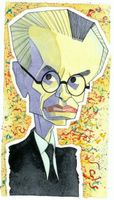Mar 25, 2005

godel part 6 - completion

Godel links: Part 1 | Part 2 | Part 3 | Part 4 | Part 5 | Part 6 | Part 7Let’s finish up. Note this is going to go a lot quicker than you expect. We've really done the hard stuff in the previous posts. Godel's genius really lies in the Provability arithmetic property and his Godel numbering scheme that allowed that property to exist. Don't underestimate what he did here. Developing those two parts of this theorem encapsulate the hardest parts of the theorem. Everything that follows is really quite straightforward. I may at some point revisit both those topics and delve in a little more deeply. But for now let's just get to the end. Let’s start again with Godel’s Pr(x) property. Remember that a natural number will have the arithmetical property Pr(x) if and only if it corresponds to a provable proposition under the Godel numbering. Again,
Pr(GN(p)) is true if and only if p is a provable proposition
Now let’s look at the property
~ Pr(x)
Remember the “`” is a negation symbol. This second property will be true of all Godel numbers which correspond to non-theorems of the system. In other words
~Pr(GN(p)) is true if and only if p is not a provable proposition (1)
Keep in mind here that what I’ve written is neither an arithmetical sentence nor a sentence of the formal system. It is really a meta-mathematical statement; a statement about statements within the formal system. Now let’s add in the diagonal lemma where our previous F(x) = ~Pr(x). We know from this lemma that there is some number (let’s call it g) such that
g = GN(~Pr(g)) (2)
Or g is the Godel number of the proposition that states that the number g lacks the arithmetical property Pr. Clearly we have set up a self referential statement here. g is talking about itself. Let’s frame our proposition G now
G = ~Pr(g)
In other words G states that the number g is not provable. But g is a Godel number of a proposition. So really we have written that G states that the proposition with a Godel number g is not provable. Look at the two previous statements we can write
g = GN(G)
g is the Godel number of the proposition G. Let’s go back to (1) and rewrite
~Pr(GN(p)) is true if and only if p is not provable
Letting p = G and using our previous equations we get,
~Pr(g) is true if and only if G is not provable
or
G is true if and only if G is not provable
And there we have it. It’s saying that G is not provable. Is G true? It couldn’t be false because then it would be provable and thus it would be true anyway; unless of course the formal system is contradictory or non-consistent. Keep in mind that we haven’t shown something to be true and unprovable inside the system here. We have shown that G is true by showing that it can’t be proved (just as it states).

Once again. Godel has shown that there are provably improvable propositions that are nonetheless true in a formal system that describes formal arithmetic assuming it is consistent. The top level point here is that you can’t have your cake and eat it too. Make a system that is fully consistent to describe arithmetic and you are going to have some theorems that cannot be proved. Or prove everything but also show two contradictory statements are true somewhere in the system.

That’s all it says. That is Godel’s 1st Incompleteness Theorem. This first theorem leads to a second theorem that we will discuss next. And that theorem leads to all sorts of speculation about the human mind, artificial intelligence, and philosophy in general. We will finish our Godel week with that.

I will finish this post with a comment that Godel always had high aspirations regarding his mathematical work. He in fact wanted to develop mathematics that transcended math; math that spoke about mathematics, philosophy, and ultimately the existence of God. It is quite a feat that he at least reached his first goal.